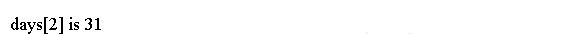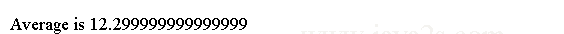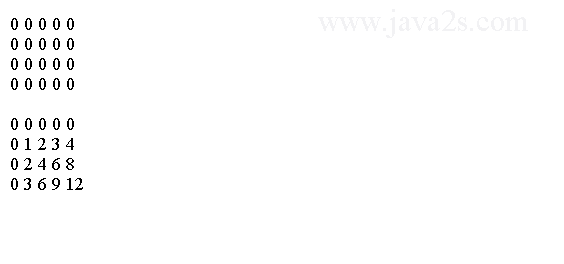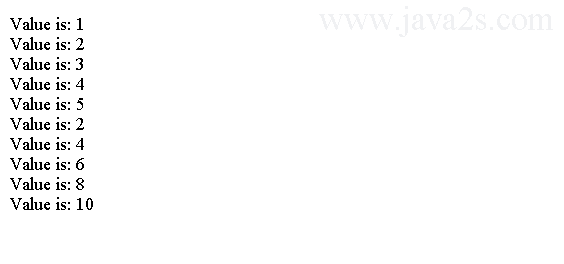# Java Tutorial - Java Array

An array is a named set of variables of the same type.

Each variable in the array is called an array element.

## Java Array Variables

A Java array variable has two parts: array type and array object.

The array type is the type of the array variable. The array object is the memory allocated for an array variable.

When defining an array we can first define the array type and allocate the memory later.

## Syntax

You could declare the integer array variable myIntArray with the following statement:

```int[] myIntArray;                // Declare an integer array variable

```

The variable myIntArray is now a type for an integer array. No memory has been allocated to hold an array itself.

Later we will create the array by allocating memory and specify how many elements it can contain.

The square brackets following the type indicates that the variable is for an array of int values, and not for storing a single value of type int.

The type of the array variable is int[].

## Alternative Syntax

We can use an alternative notation for declaring an array variable:

```int myIntArray[];                // Declare an integer array variable

```

Here the square brackets appear after the variable name, rather than after the type name.

This is exactly equivalent to the previous statement. int[] form is preferred since it indicates more clearly that the type is an array of values of type int.

The following two declarations are equivalent:

```int a1[] = new int;
int[] a2 = new int;
```

## Array create

After you have declared an array variable, you can define an array that it references:

```myIntArray = new int;        // Define an array of 10 integers
```

This statement creates an array that stores 10 values of type int and stores a reference to the array in the variable `myIntArray`.

The reference is simply where the array is in memory.

You could also declare the array variable and define the array of type int to hold 10 integers with a single statement.

```int[] myIntArray = new int;            //An array of 10 integers
```

The first part of the definition specifies the type of the array. The element type name, int in this case, is followed by an empty pair of square brackets.

The part of the statement that follows the equal sign defines the array.

The keyword new indicates that you are allocating new memory for the array, and int specifies that the capacity is 10 variables of type int in the array.

## Java Array Initial Values

After we allocate memory for a Java array, Java assigns each element in an array to its initial values

The initial value is zero in the case of an array of numerical values, is false for boolean arrays, is '\u0000' for arrays storing type char, and is null for an array of objects of a class type.

The following table lists the default value for various array types.

Element Type Initial Value
byte 0
int 0
float 0.0f
char '\u0000'
object referencenull
short 0
long 0L
double 0.0d
boolean false

## Java Array Length

You can refer to the length of the array - the number of elements it contains - using length, a data member of the array object.

Array size, `arrayName.length`, holds its length.

The following code outputs the length of each array by using its `length` property.

```public class Main {
public static void main(String args[]) {
int a1[] = new int;
int a2[] = {1, 2, 3, 4, 5};
int a3[] = {4, 3, 2, 1};
System.out.println("length of a1 is " + a1.length);
System.out.println("length of a2 is " + a2.length);
System.out.println("length of a3 is " + a3.length);
}/*from  www.ja  va  2  s.com*/
}
```

This program displays the following output:## Java Initialize Arrays

We can initialize the elements in an array with values when declaring it, and at the same time determine how many elements it has.

To do this, we simply add an equal sign followed by the list of element values enclosed between braces following the specification of the array variable.

For example, you can define and initialize an array with the following statement:

```int[] primes = {2, 3, 5, 7, 11, 13, 17};    // An array of 7 elements
```

The array size is determined by the number of initial values.

The values are assigned to the array elements in sequence, so in this example primes has the initial value 2, primes has the initial value 3, primes has the initial value 5, and so on through the rest of the elements in the array.

When an array is initialized during the declaration there is no need to use new.

```public class Main {
public static void main(String args[]) {

int days[] = {31, 28, 31,};
System.out.println("days is " + days);
}
}
```

The output:## Java Array Index

To reference an element in an array, we use the array name combined with an integer value, called index.

The index is placed between square brackets following the array name; for example,

`data `

refers to the element in the data array corresponding to the index value 9.

The index for an array element is the offset of the element from the beginning of the array.

The first element has an index of 0, the second has an index of 1, the third an index of 2, and so on.

We refer to the first element of the myIntArray array as myIntArray, and we reference the fifth element in the array as myIntArray.

data refers to the tenth element in the data array.

The maximum index value for an array is one less than the number of elements in the array.

The index value does not need to be an integer literal, it can also be a variable.

The array index has to have a value equals to or greater than zero.

Array stores elements and we use index to reference a single value in an array. The starting value of the index is 0. If you try to reference elements with negative numbers or numbers greater than the array length, you will get a run-time error.

```public class Main {
public static void main(String args[]) {

int days[] = {1, 2, 3,};
System.out.println("days is " + days);
}
}
```

It generates the following error.## for loop

We usually use a for loop to access each element in an array. The following code uses a one-dimensional array to find the average of a set of numbers.

```public class Main {
public static void main(String args[]) {
double nums[] = {10.1, 11.2, 12.3, 13.4, 14.5};
double result = 0;
int i;//from  w  w w .ja v a 2 s  . co m

for (i = 0; i < 5; i++)
result = result + nums[i];

System.out.println("Average is " + result / 5);
}
}
```

The output:## Java Array for each loop

We can use a collection-based for loop as an alternative to the numerical for loop when processing the values of all the elements in an array.

The syntax of for each loop for an array is as follows.

```for(arrayType variableName: array){
process each variableName
}
```

Java array for each loop

```public class Main {
public static void main(String args[]) {
int days[] = {1, 2, 3,};
for(int i:days){
System.out.println(i);
}
}
}
```

The code above generates the following result.## Java Multidimensional Arrays

In Java, multidimensional arrays are actually arrays of arrays.

For example, the following declares a two-dimensional array variable called `twoD`.

```int twoD[][] = new int;
```

This allocates a 4-by-5 array and assigns it to twoD. This array will look like the one shown in the following:

```   [leftIndex][rightIndex]

    
    
    
    
```

The wrong way to think about multi-dimension arrays is as follows.

```+----+----+----+
|   1|   2|   3|
+----+----+----+
|   4|   5|   6|
+----+----+----+
|   7|   8|   9|
+----+----+----+
```

The right way to think about multi-dimension arrays

```+--+        +----+----+----+
|  |--------|   1|   2|   3|
+--+        +----+----+----+     +----+----+----+
|  |-----------------------------|   4|   5|   6|
+--+   +----+----+----+          +----+----+----+
|  |---|   7|   8|   9|
+--+   +----+----+----+
```

The following code use nested for loop to assign values to a two-dimensional array.

```public class Main {
public static void main(String args[]) {
int twoD[][] = new int;
for (int i = 0; i < 4; i++) {
for (int j = 0; j < 5; j++) {
twoD[i][j] = i*j;/*from www .ja v a  2s.  c  om*/
}
}
for (int i = 0; i < 4; i++) {
for (int j = 0; j < 5; j++) {
System.out.print(twoD[i][j] + " ");
}
System.out.println();
}
}
}
```

This program generates the following output:## Example

The following program creates a 3 by 4 by 5, three-dimensional array.

```public class Main {
public static void main(String args[]) {
int threeD[][][] = new int;
//from w  w w. j a v a 2  s . c o m
for (int i = 0; i < 3; i++)
for (int j = 0; j < 4; j++)
for (int k = 0; k < 5; k++)
threeD[i][j][k] = i * j * k;

for (int i = 0; i < 3; i++) {
for (int j = 0; j < 4; j++) {
for (int k = 0; k < 5; k++)
System.out.print(threeD[i][j][k] + " ");
System.out.println();
}
System.out.println();
}
}
}
```

This program generates the following output:## Example 2

The following code shows how to iterate over Multidimensional Arrays with for-each.

```public class Main {
public static void main(String args[]) {
int sum = 0;//www . j  a  va2 s.  c o m
int nums[][] = new int;

// give nums some values
for (int i = 0; i < 3; i++)
for (int j = 0; j < 5; j++)
nums[i][j] = (i + 1) * (j + 1);

// use for-each for to display and sum the values
for (int x[] : nums) {
for (int y : x) {
System.out.println("Value is: " + y);
sum += y;
}
}
System.out.println("Summation: " + sum);
}
}
```

The code above generates the following result.## Jagged array

When you allocate memory for a multidimensional array, you can allocate the remaining dimensions separately.

An irregular multi-dimension array

```+--+        +----+----+
|  |--------|   1|   2|
+--+        +----+----+          +----+----+----+
|  |-----------------------------|   4|   5|   6|
+--+   +----+----+----+----+     +----+----+----+
|  |---|   7|   8|   9|  10|
+--+   +----+----+----+----+
```

For example, the following code allocates the second dimension manually.

```public class Main {
public static void main(String[] argv) {
int twoD[][] = new int[];
twoD = new int;
twoD = new int;
twoD = new int;
twoD = new int;
}
}
```

When allocating dimensions manually, you do not need to allocate the same number of elements for each dimension.

## Example 3

The following program creates a two-dimensional array in which the sizes of the second dimension are unequal.

```public class Main {
public static void main(String args[]) {
int twoD[][] = new int[];
twoD = new int;
twoD = new int;
twoD = new int;
twoD = new int;
/*from  w w w. j  ava2s .  c  om*/
for (int i = 0; i < 4; i++){
for (int j = 0; j < i + 1; j++) {
twoD[i][j] = i + j;
}
}
for (int i = 0; i < 4; i++) {
for (int j = 0; j < i + 1; j++)
System.out.print(twoD[i][j] + " ");
System.out.println();
}
}
}
```

This program generates the following output:The array created by this program looks like this:## Example 4

We can initialize multidimensional arrays during declaration by enclosing each dimension's initializer within its own set of curly braces.

```public class Main{
public static void main(String args[]) {
double m[][] = {
{ 0, 1, 2, 3 }, //from   ww w .  j  ava  2s .c om
{ 0, 1, 2, 3 },
{ 0, 1, 2, 3 },
{ 0, 1, 2, 3 }
};
for(int i=0; i<4; i++) {
for(int j=0; j<4; j++){
System.out.print(m[i][j] + " ");
}
System.out.println();
}
}
}
```

When you run this program, you will get the following output: